ConceptDraw
DIAGRAM 17

# Network Diagram SoftwareTopology Network

## Network Topology Diagramming

Network Topology Diagrams visualize the telecommunication and computer network topology and layout. They are used by IT professionals and corporate IT departments, network and system administrators to visually document the topology of computer networks. ConceptDraw has 1004 vector stencils in the 40 libraries that helps you to start using software for designing your own Network Topology Diagrams. You can use the appropriate stencils from Computer and Network Diagrams object libraries.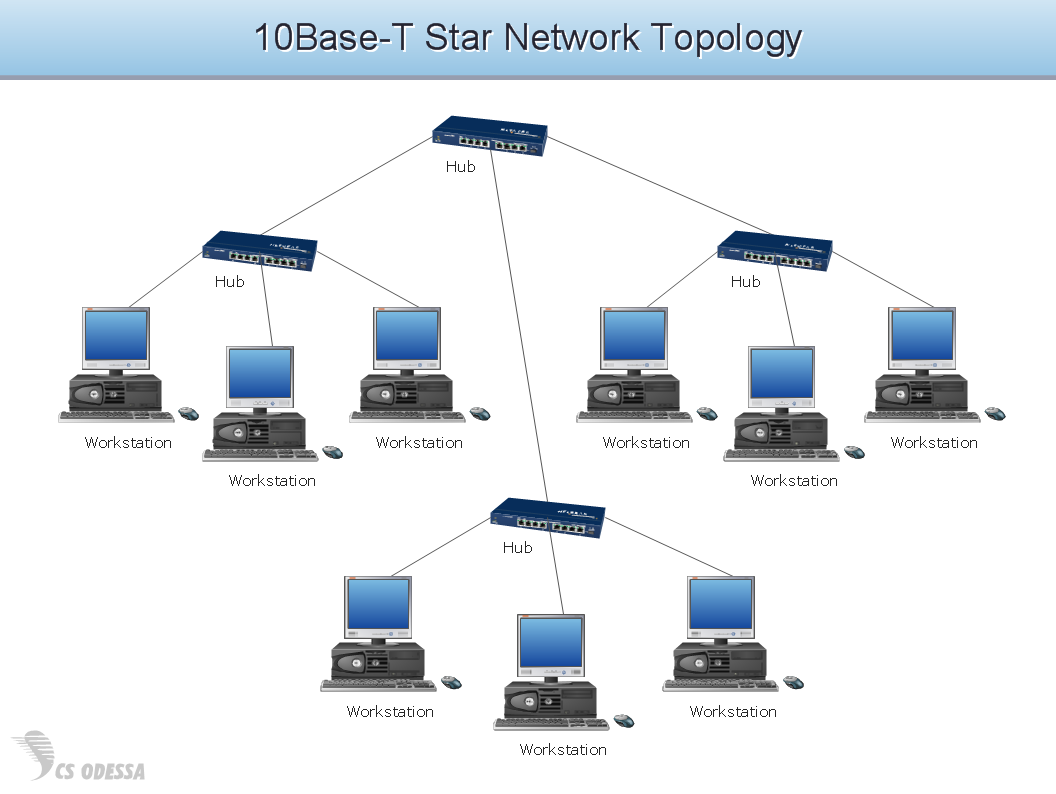This network topology diagram sample is created using ConceptDraw DIAGRAM diagramming and vector drawing software enhanced with Computer & Networks solution from ConceptDraw Solution Park.

Computer & Networks solution provides samples, templates and libraries of symbols for all types of computer network diagrams, LAN and WAN floor plan layouts, network communication plans, Cisco network diagrams, Active Directory diagrams, logical and physical network diagrams, network architecture and topology diagrams.

### Tree Network Topology Diagram →

When designing a network, it is important to take into account the hierarchy of network devices. When you have a root device you should consider a tree network topology that might have any number of lower levels as you might need. This technology is based on star and bus network topologies. This is a schematic representation of a Tree computer network topology. A tree topology means that some star networks are linked together. A star network is a topology of the local network where a central workstation is connected with each end-user computer or peripherals. A tree structure means that, the central nodes of these star networks are linked to a main cable (the Bus topology). So, a Tree network topology is a few Star networks connected into a Bus topology. This scheme can be applied to draw the particular physical or logical network diagrams using the ConceptDraw Computer and Networks solution.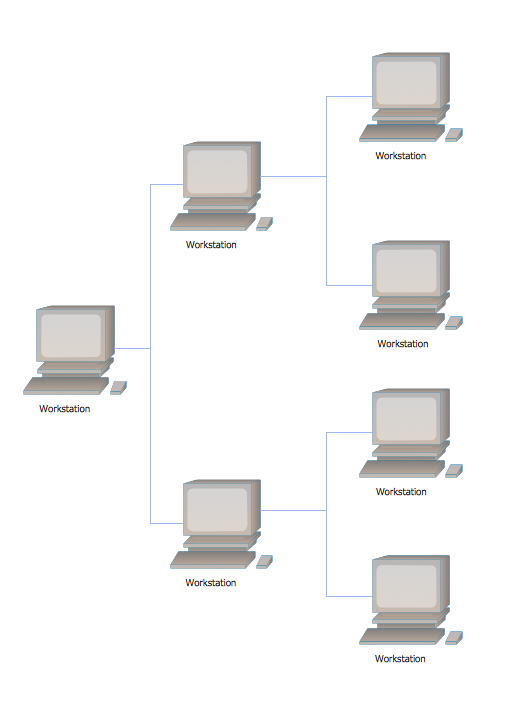Picture: Tree Network Topology Diagram
Related Solution:

### Cisco Network Diagram Software →

ConceptDraw DIAGRAM is the best network diagram software. To design the Cisco Network Diagrams and visualize the computer networks topology use ConceptDraw DIAGRAM diagramming and vector drawing software extended with Cisco Network Diagrams solution for ConceptDraw DIAGRAM Solution Park.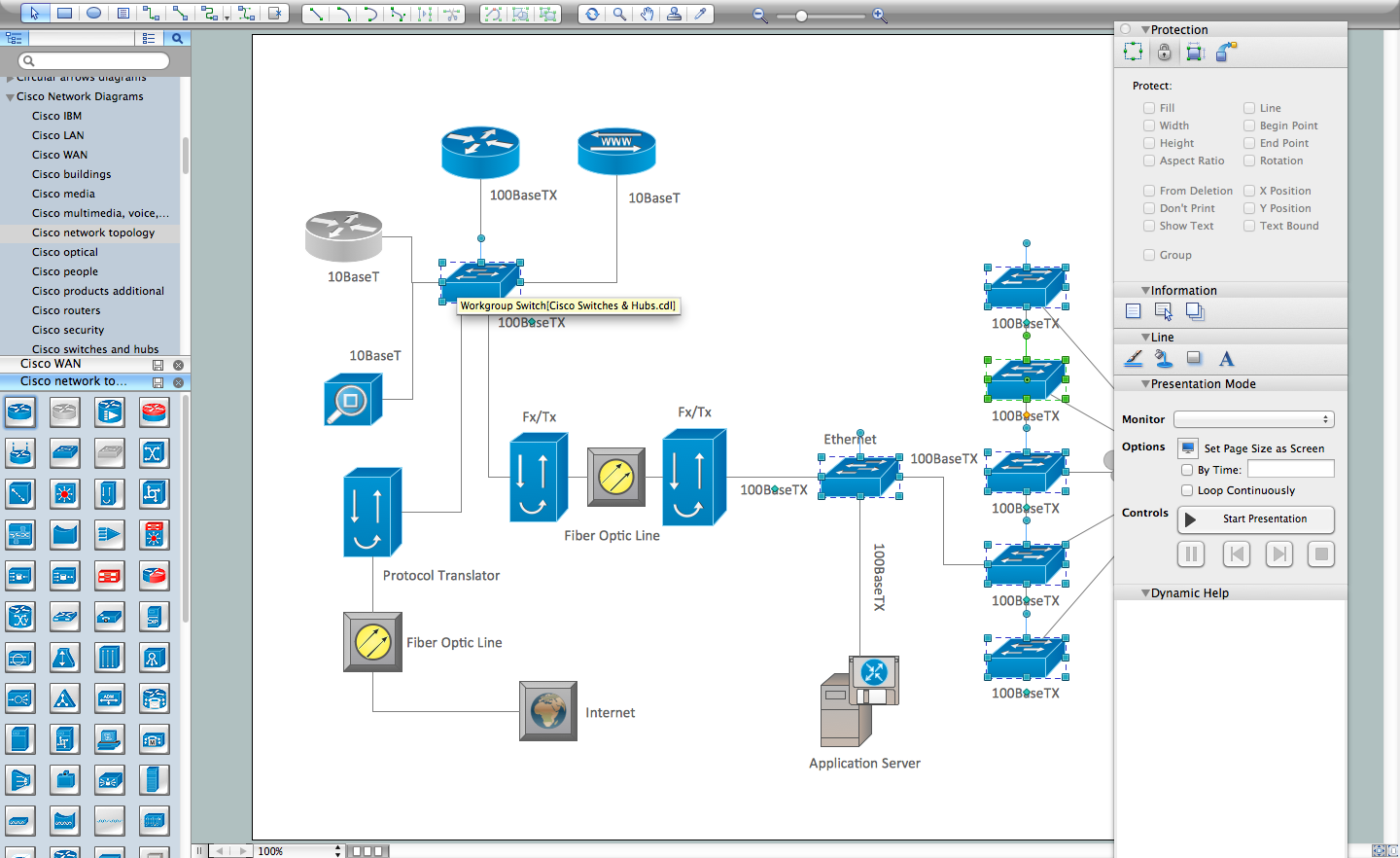Picture: Cisco Network Diagram Software
Related Solution:

### Cisco Network Examples and Templates →

The Cisco Network Diagrams solution from the Computer and Networks area of ConceptDraw Solution Park provides a wide collection of predesigned templates and samples that help you to create the Cisco Network Diagrams in one moment.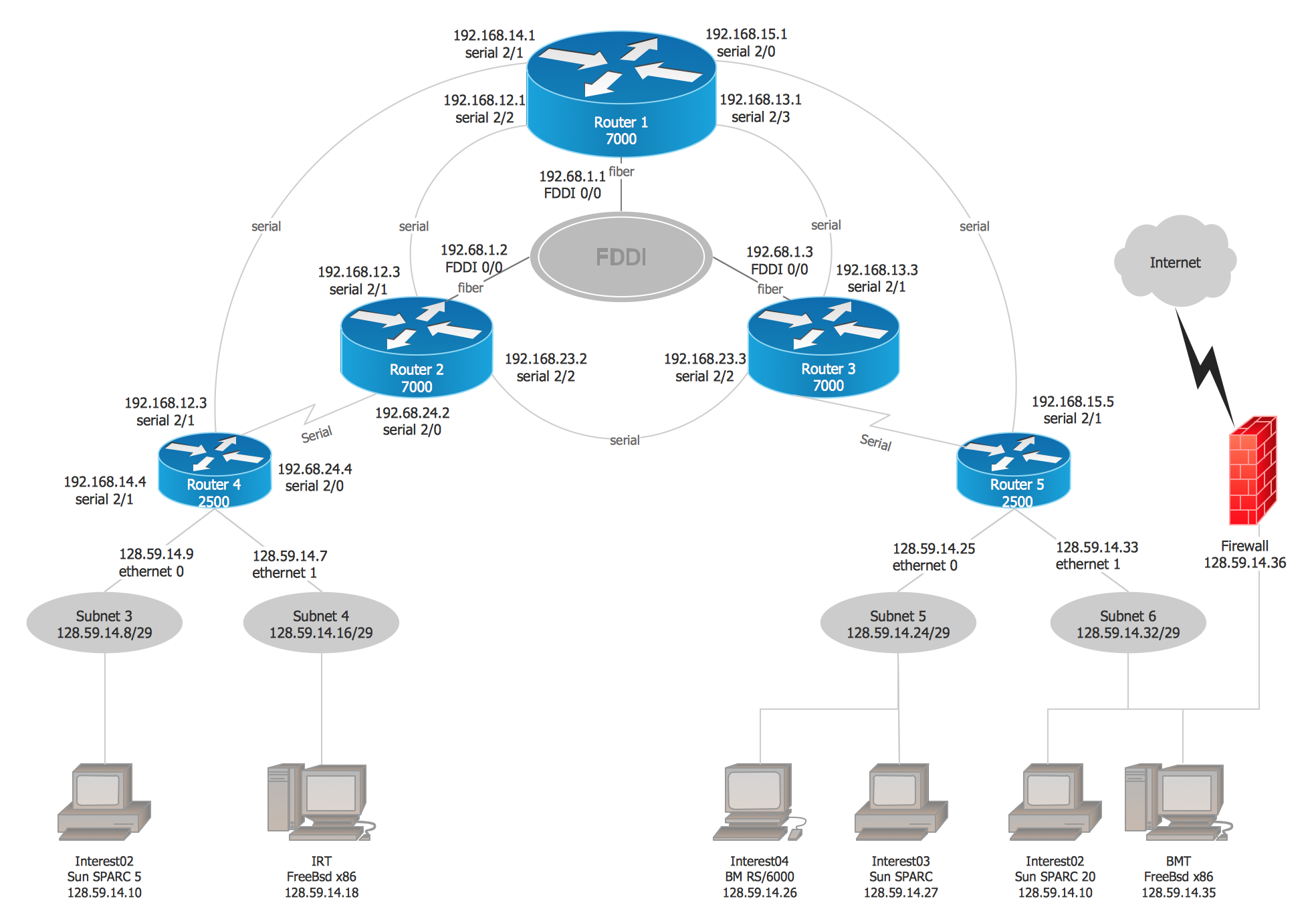Picture: Cisco Network Examples and Templates
Related Solution:

### Mesh Network. Computer and Network Examples →

A Mesh Topology is a computer network topology that is based on the cells concept, in which each workstation connects with several other workstations of the same network with the possible to accept the switch functions for other workstations. This example was created in ConceptDraw DIAGRAM using the Computer and Networks solution from the Computer and Networks area of ConceptDraw Solution Park and shows the Mesh Network.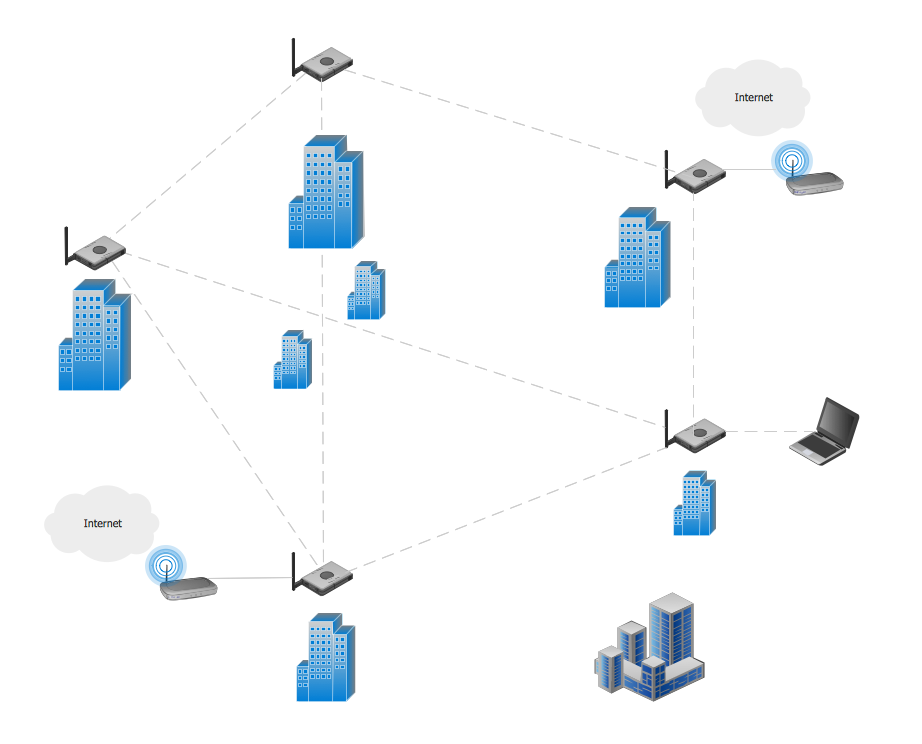Picture: Mesh Network. Computer and Network Examples
Related Solution:

### Hierarchical Network Topology →

This sample was created in ConceptDraw DIAGRAM diagramming and vector drawing software using the Computer and Networks solution from Computer and Networks area of ConceptDraw Solution Park. This sample shows the Hierarchical network topology. A Hierarchical network topology interconnects multiple groups that are located on the separate layers to form a larger network. Each layer concentrates on the specified functions, this allows to choose the right equipment for the layer.Picture: Hierarchical Network Topology
Related Solution:

### Probability Quality Control Tools →

The Total Quality Management Diagram solution helps your organization visualize business and industrial processes. Create Total Quality Management diagrams for business process with ConceptDraw software.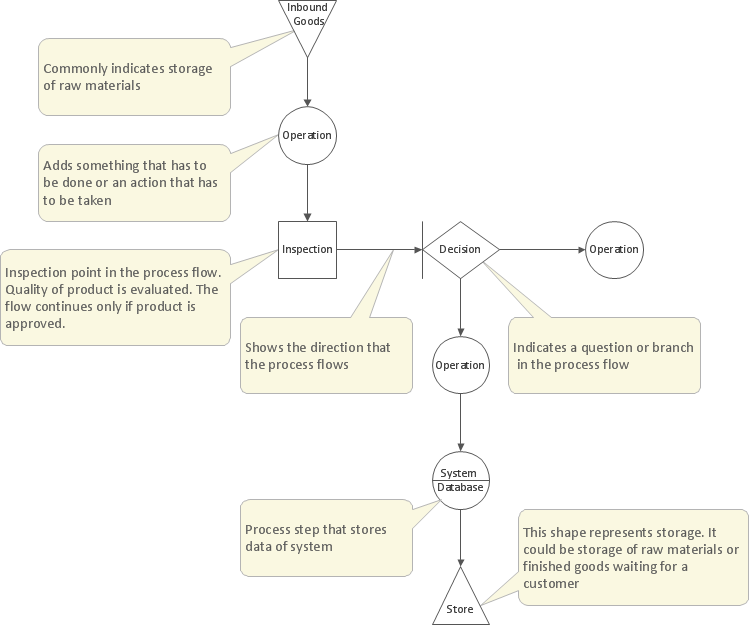Picture: Probability Quality Control Tools
Related Solution:
ConceptDraw
DIAGRAM 17